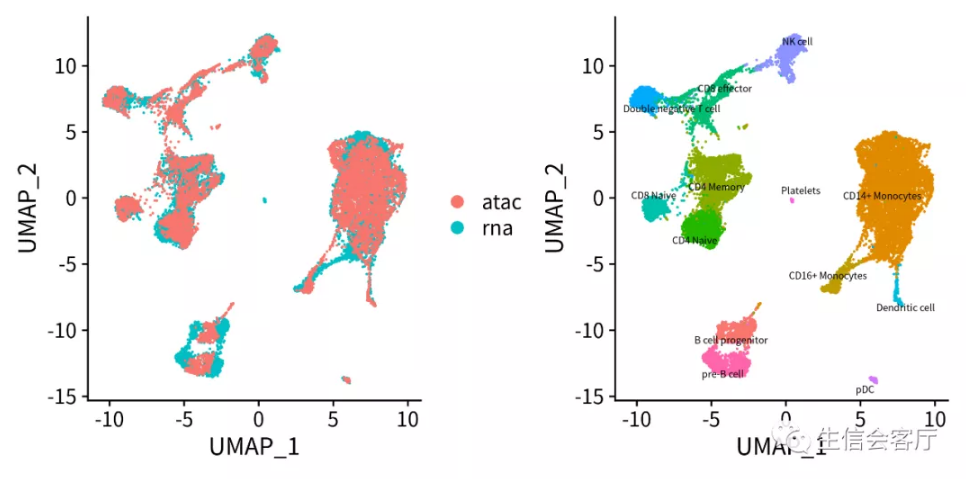# scATAC分析

## 1. scATAC简介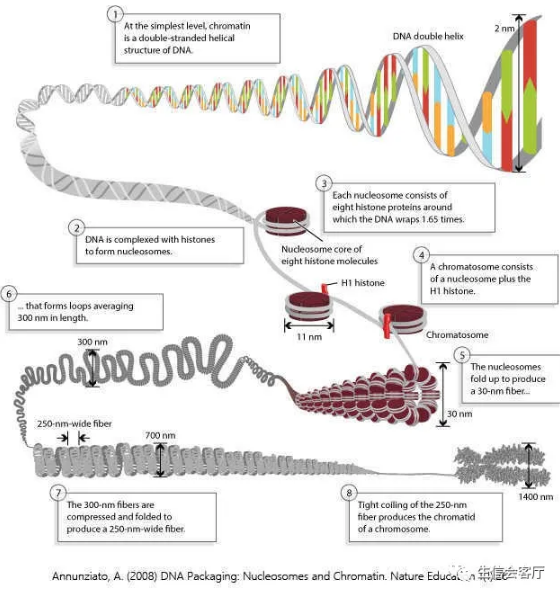2013年美国斯坦福大学的William Greenleaf教授研发了一种全新的方法，利用DNA转座酶结合高通量测序技术来研究染色体的可及性，即ATAC-seq (Assay for transposase-accessible chromatin with high-throughput sequencing)。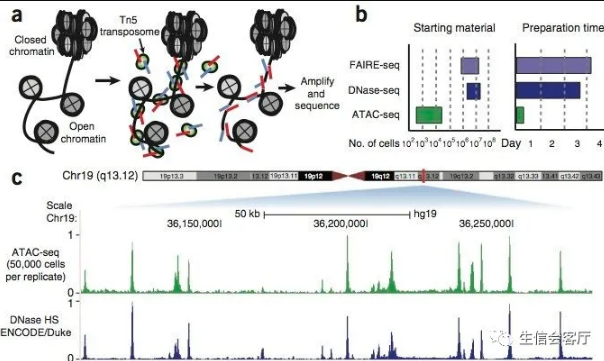DNA转座，是一种把DNA序列从染色体的一个区域搬运到另外一个区域的现象，由DNA转座酶来实现。这种转座插入DNA，也是需要插入位点的染色质是开放的，否则就会被一大坨高级结构给卡住。那么，如上图a，我们只要人为地，将携带已知DNA序列标签的转座复合物（即带着红色蓝色测序标签的转座酶Tn5），加入到细胞核中，再利用已知序列的标签进行PCR后测序，就知道哪些区域是开放染色质了。而这也就是ATAC-seq的原理。

## 2. 10X scATAC-seq的简介

10X Genomics公司的scATAC-seq是目前主流的单细胞水平染色质可及性测序解决方案，国内已经有很多公司推出了商业化服务。该技术基于10X Genomics Chromium平台，可用于绘制细胞染色质开放区的单细胞图谱，是一种单细胞水平研究表观遗传学的有效手段。其工作流程如下：

• 使用改造过的Tn5转座酶孵育细胞核，Tn5转座酶剪切开放区域DNA并加上测序接头；
• 利用微流控系统将带有barcode引物的凝胶微珠和单个细胞核包裹在油滴中；
• 油滴内凝胶微珠溶解释放出带barcode的引物，细胞核裂解释放带有测序接头的染色质开放区域DNA，引物与DNA结合进行PCR扩增；
• 油层破裂，DNA片段释放出来，进行后续的Illumina文库构建和测序。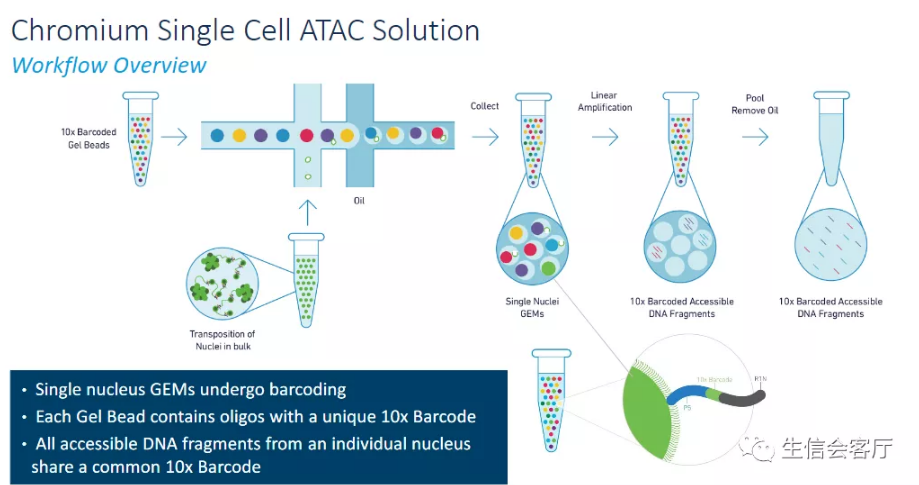## 3. scATAC-seq的意义

• 在单细胞水平揭示细胞核内染色质可及性；
• 揭示染色质可及性在细胞间的异质性；
• 鉴定特定细胞类型的染色质活性区域；

## 4. scRNA与scATAC的整合分析

DNA层面的调控与mRNA层面的表达存在因果关系，因此整合两种类型的数据既可以相互印证研究结论，也更容易找到完整的调控网络环路。此外，在疾病研究中使用多组学数据基于主效应或交互作用筛选生物标志物可提高疾病预后的准确性。

### 4.0 下载分析数据

scATAC-seq peak矩阵 http://cf.10xgenomics.com/samples/cell-atac/1.0.1/atac_v1_pbmc_10k/atac_v1_pbmc_10k_filtered_peak_bc_matrix.h5
scRNA-seq seurat对象 https://www.dropbox.com/s/3f3p5nxrn5b3y4y/pbmc_10k_v3.rds?dl=1
GRCh37基因组注释文件 ftp://ftp.ensembl.org/pub/grch37/release-84/gtf/homo_sapiens/Homo_sapiens.GRCh37.82.gtf.gz

scRNA-seq seurat对象文件是降维聚类并鉴定细胞类型的scRNA数据，下载困难的朋友可以下载官方的表达矩阵自己处理。 scRNA-seq 表达矩阵 http://cf.10xgenomics.com/samples/cell-exp/3.0.0/pbmc_10k_v3/pbmc_10k_v3_filtered_feature_bc_matrix.h5

### 4.1 scATAC数据预处理

``````library(Seurat)
library(ggplot2)
library(rtracklayer)
library(patchwork)
rm(list=ls())
dir.create("ATAC")
set.seed(811)
``````

scATAC-seq得到的初始文件是peak矩阵，行为染色体坐标，列为细胞barcode，这些染色体坐标代表染色质可及性区域。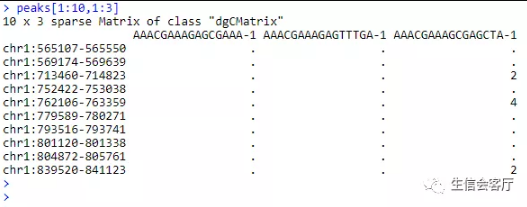``````activity.matrix <- CreateGeneActivityMatrix(peak.matrix = peaks,
annotation.file = "Data/ATAC/Homo_sapiens.GRCh37.82.gtf",
seq.levels = c(1:22, "X", "Y"), upstream = 2000, verbose = TRUE)
``````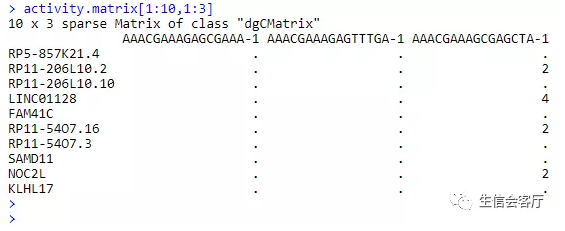``````pbmc.atac <- CreateSeuratObject(counts = peaks, assay = "ATAC", project = "10x_ATAC")
pbmc.atac[["ACTIVITY"]] <- CreateAssayObject(counts = activity.matrix)
meta <- read.table("Data/ATAC/atac_v1_pbmc_10k_singlecell.csv", sep = ",",
header = TRUE, row.names = 1, stringsAsFactors = FALSE)
meta <- meta[colnames(pbmc.atac), ]
pbmc.atac <- subset(pbmc.atac, subset = nCount_ATAC > 5000)
pbmc.atac\$tech <- "atac"
``````

``````#对活性矩阵执行标准化与中心化处理
DefaultAssay(pbmc.atac) <- "ACTIVITY"
pbmc.atac <- FindVariableFeatures(pbmc.atac)
pbmc.atac <- NormalizeData(pbmc.atac)
pbmc.atac <- ScaleData(pbmc.atac)
#对peak矩阵降维
DefaultAssay(pbmc.atac) <- "ATAC"
VariableFeatures(pbmc.atac) <- names(which(Matrix::rowSums(pbmc.atac) > 100))
pbmc.atac <- RunLSI(pbmc.atac, n = 50, scale.max = NULL)
pbmc.atac <- RunUMAP(pbmc.atac, reduction = "lsi", dims = 1:50)
``````

### 4.2 整合前scRNA与scATAC数据展示

``````pbmc.rna <- readRDS("Data/ATAC/pbmc_10k_v3.rds")
pbmc.rna\$tech <- "rna"
#展示整合前的scATAC和scRNA数据
p1 <- DimPlot(pbmc.atac, reduction = "umap") + NoLegend() + ggtitle("scATAC-seq")
p2 <- DimPlot(pbmc.rna, group.by="celltype", label=TRUE, label.size=2,
repel = TRUE) + NoLegend() + ggtitle("scRNA-seq")
plotc = p1 + p2
ggsave('ATAC/ATAC-RNA_beforeINT.png', plotc, width = 8, height = 4)
``````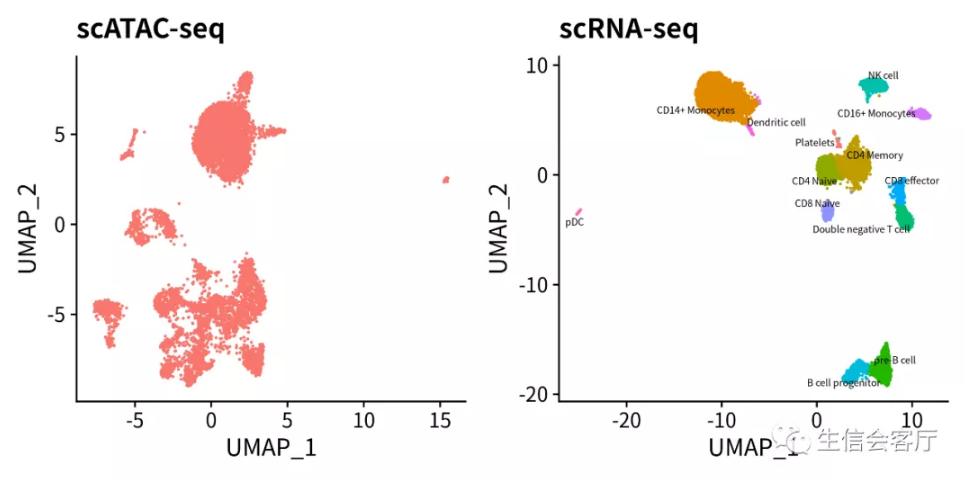### 4.3 识别scATAC数据集的细胞类型

``````#通过锚点建立数据集之间的配对关系
transfer.anchors <- FindTransferAnchors(reference=pbmc.rna, query = pbmc.atac,
features=VariableFeatures(object=pbmc.rna), reference.assay = "RNA",
query.assay = "ACTIVITY", reduction = "cca")
#数据迁移
celltype.predictions <- TransferData(anchorset = transfer.anchors, refdata = pbmc.rna\$celltype,
weight.reduction = pbmc.atac[["lsi"]])
#展示id转换后的scATAC和scRNA数据
pbmc.atac.filtered <- subset(pbmc.atac, subset = prediction.score.max > 0.5)
pbmc.atac.filtered\$predicted.id <- factor(pbmc.atac.filtered\$predicted.id, levels=levels(pbmc.rna))
p1 <- DimPlot(pbmc.atac.filtered, group.by="predicted.id", label=TRUE, label.size=2, repel=TRUE) +
ggtitle("scATAC-seq cells") + NoLegend() + scale_colour_hue(drop = FALSE)
p2 <- DimPlot(pbmc.rna, group.by="celltype", label=TRUE, label.size=2, repel=TRUE) +
ggtitle("scRNA-seq cells") + NoLegend()
plotc = p1 + p2
ggsave('ATAC/ATAC-RNA_transfer.png', plotc, width = 8, height = 4)
``````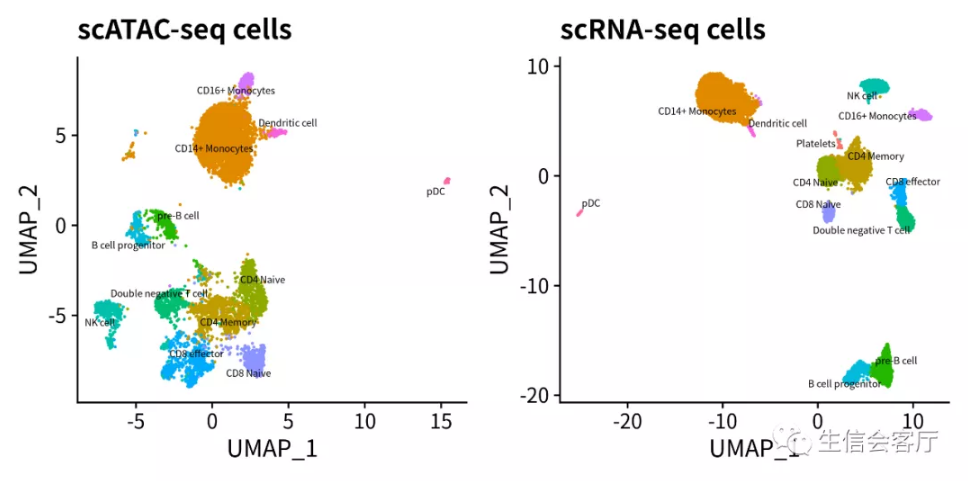### 4.4 观察两个数据集的异同

``````#将scRNA细胞的转录谱通过锚点转移给scATAC细胞
genes.use <- VariableFeatures(pbmc.rna)
refdata <- GetAssayData(pbmc.rna, assay="RNA", slot="data")[genes.use, ]
imputation <- TransferData(anchorset=transfer.anchors, refdata=refdata, weight.reduction = pbmc.atac[["lsi"]])
pbmc.atac[["RNA"]] <- imputation
#合并两个数据集的seurat对象
coembed <- merge(x = pbmc.rna, y = pbmc.atac)
#合并后的数据集执行降维分析
coembed <- ScaleData(coembed, features = genes.use, do.scale = FALSE)
coembed <- RunPCA(coembed, features = genes.use, verbose = FALSE)
coembed <- RunUMAP(coembed, dims = 1:30)
coembed\$celltype <- ifelse(!is.na(coembed\$celltype), coembed\$celltype, coembed\$predicted.id)
p1 <- DimPlot(coembed, group.by = "tech")
p2 <- DimPlot(coembed, group.by="celltype", label=TRUE, label.size=2, repel=TRUE) + NoLegend()
plotc = p1 + p2
ggsave('ATAC/ATAC-RNA_coembed.png', plotc, width = 8, height = 4)
``````